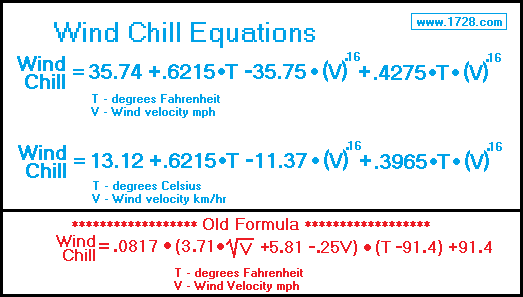Wind Chill CalculatorIn the winter, with the temperature remaining the same, it always feels much colder when the wind is blowing than if there were no wind. This cooling effect is called the wind chill factor.

Why does this occur? Your body generates heat, producing a small layer of warm air near your skin. When the wind blows, this layer of warm air is easily removed, causing you to feel much colder.

Scientists have studied this phenomenon in an attempt to determine how much cooling the wind produces and they were able to derive a formula which could calculate the wind chill temperature. (See old formula). Eventually, it was discovered that this formula was somewhat inaccurate and additional research produced a new formula which has been in use since 2001.

Basically, the old formula calculated the wind chill factor to be much colder than it actually is. For a quick example, using the calculator, determine the wind chill for 20 degrees Fahrenheit with a 30 miles per hour wind. The calculator shows the wind chill to be 1.3℉ but the old formula calculates it as -17.3℉ - an 18 degree difference!

Significant Figures
The default numeric display is 3 significant figures.
For easier readability, numbers that are greater than .001 and less than 1000 are NOT in scientific notation.
You can change the display option by changing the number in the box above.
Entering a zero eliminates all formatting.

"R E T U R N"